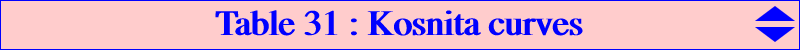The Kosnita triangle is formed by the circumcenters Oa, Ob, Oc of the three triangles OBC, OCA, OAB. It is homothetic to several triangles namely the orthic, tangential, extangents triangles for example. Now, let P be a point in the plane of the reference triangle ABC and denote by cevP : the cevian triangle of P acvP : the anticevian triangle of P pedP : the pedal triangle of P apdP : the antipedal triangle of P ccvP : the circumcevian triangle of P cacP : the circumanticevian triangle of P. We seek the locus of P such that the Kosnita triangle is perspective, orthologic, parallelogic to one of these triangles. The following table gives these loci. L denotes the line at infinity, C denotes the circumcircle. Obviously, concerning orthologic and parallelogic triangles, the Kosnita triangle can be replaced by any homothetic triangle.triangle perspective orthologic parallelogic cevP pK(X2, X311) (*) Euler perspector cubic K045 nK(X4, X264) = K211 acvP pK(X571, X1994) (*) K612 = pK(X216, X2) circum-cubic K0 pedP a K+ L + Euler line L + line X186-X523 = tripolar of X275 apdP Jerabek strophoid K039 L + C + Jerabek hyperbola L + C + circum-conic with center X(5) through X110, X265, X1625 ccvP L + C + Napoleon cubic K005 C + quartic through X186 cacP isogonal sextic sextic through X2, X6 circum-sextic(*) in both cases, the locus of the perspector is K388.